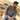How to loop through an array in JavaScriptTaimoor SattarThere are different ways to loop in an array using JavaScript. In JavaScript, we use the loop to iterate over an array. We will discuss two methods in JavaScript to loop in an array as below:

• Array forEach method

let’s define an array as below:

let list = [1, 2, 3, "one", "two", "three"];

We can perform loop in array using traditional loop method in JavaScript as below:

for(let i = 0; i < list.length; i++){
console.log(list[i]);
}

Moreover, you can mention breaking statements in the loop to exit out of the loop if the condition is true. See the example below:

for(let i = 0; i < list.length; i++){
if(list[i] == 3) break; // If true, loop will break
console.log(list[i]);
}

Using Array forEach method, we can perform loop as below:

list.forEach(function(ele) {
console.log(ele);
});

What one to prefer?

I prefer to use the traditional loop method instead of foreach loop method for the following reasons.

• foreach does not support break statements. You have to add additional checks in the foreach loop to create a break statement.
• Performance of the foreach loop is not good as compare to than traditional loop in Javascript